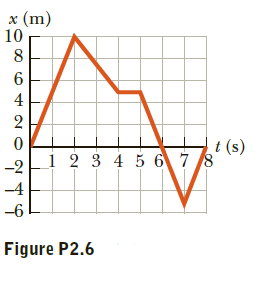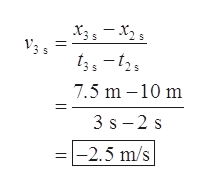# х (m)108t (s)_i 2 3 4 5 678-2-4-6Figure P2.66420

Question
8 views

A graph of position versus time for a certain particle moving
along the x - axis is shown in Figure P2.6. Find the instantaneous
velocity at the instants (a) t = 1.00 s, (b) t = 3.00 s,
(c) t = 4.50 s, and (d) t = 7.50 s.help_outlineImage Transcriptioncloseх (m) 10 8 t (s) _i 2 3 4 5 678 -2 -4 -6 Figure P2.6 6420 fullscreen
check_circle

Step 1

Instantaneous velocity of a particle is equivalent to the slope of the distance time graph.  The expression for the Instantaneous velocity is:

Step 2

(a)

Take the points at t = 0 s and t = 1 s, the plot is straight line. So, these two points can be taken in order to find the slop of the graph that is the instantaneous velocity at time t = 1 s.

Step 3

(b)

Take the points at t = 2 s and t = 3 s, the plot is straight line. So, these two points can be taken in order...help_outlineImage TranscriptioncloseX35 - X25 7.5 m – 10 m 3 s- 2 s |-2.5 m/s || fullscreen

### Want to see the full answer?

See Solution

#### Want to see this answer and more?

Solutions are written by subject experts who are available 24/7. Questions are typically answered within 1 hour.*

See Solution
*Response times may vary by subject and question.
Tagged in

### Kinematics